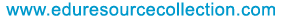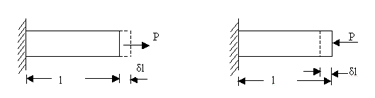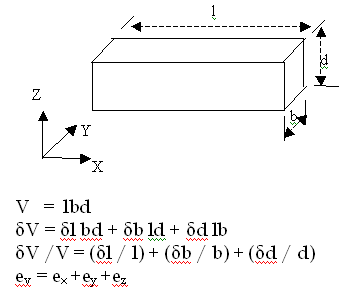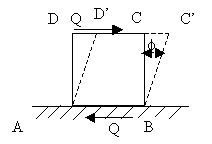@import url(http://www.google.com/cse/api/branding.css);Custom Search The content of the site is exclusively owned byHome | School Level | School Projects | Examinations | Civil | Food Processing | Seminars | Software Testing | Warehousing & Inventory | Networking

# Strength of Materials - Strains

3. Strains

Strain is defined a the ratio of change in dimension to original dimension of a body when it is deformed. It is a dimensionless quantity as it is a ratio between two quantities of same dimension.

3.1. Linear Strain
Linear strain of a deformed body is defined as the ratio of the change in length of the body due to the deformation to its original length in the direction of the force. If l is the original length and dl the change in length occurred due to the deformation, the linear strain e induced is given by e=dl/l.Linear strain may be a tensile strain, et or a compressive strain ec according as dl refers to an increase in length or a decrease in length of the body. If we consider one of these as +ve then the other should be considered as –ve, as these are opposite in nature.

3.2. Lateral Strain
Lateral strain of a deformed body is defined as the ratio of the change in length (breadth of a rectangular bar or diameter of a circular bar) of the body due to the deformation to its original length (breadth of a rectangular bar or diameter of a circular bar) in the direction perpendicular to the force.

3.3. Volumetric Strain
Volumetric strain of a deformed body is defined as the ratio of the change in volume of the body to the deformation to its original volume. If V is the original volum and dV the change in volume occurred due to the deformation, the volumetric strain ev induced is given by ev =dV/V

Consider a uniform rectangular bar of length l, breadth b and depth d as shown in figure. Its volume V is given by,This means that volumetric strain of a deformed body is the sum of the linear strains in three mutually perpendicular directions.

3.4. Shear Strain
Shear strain is defined as the strain accompanying a shearing action. It is the angle in radian measure through which the body gets distorted when subjected to an external shearing action. It is denoted by *.Consider a cube ABCD subjected to equal and opposite forces Q across the top and bottom forces AB and CD. If the bottom face is taken fixed, the cube gets distorted through angle * to the shape ABC’D’. Now strain or deformation per unit length is
Shear strain of cube = CC’ / CD = CC’ / BC = * radian